Journal of Integer Sequences, Vol. 2 (1999), Article 99.1.8

Counting Horizontally Convex Polyominoes

Dean Hickerson Dept. of Mathematics University of California, Davis Email address: dean@math.ucdavis.edu

Abstract: We present a new proof that the number, a(n), of horizontally convex n-ominoes satisfies the recurrence  a(n) = 5 a(n-1) - 7 a(n-2) + 4 a(n-3).

0. Introduction

A finite union of closed unit squares whose vertices have integer coordinates is called a polyomino if its interior is connected. A polyomino of area  n  is called an n-omino. We will consider two polyominoes to be the same if one is a translate of the other. A polyomino P is horizontally convex if each horizontal line meets P in a single line segment, or not at all. For example, the first polyomino below is horizontally convex; the second one is not.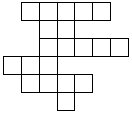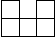Horizontally convex Not horizontally convex

Let a(n) be the number of horizontally convex n-ominoes. The table below shows the values of a(n) for  n  up to 12; see sequence A001169 in [EIS] for more values:

n  1  2  3   4   5    6    7     8     9     10     11      12
a(n)  1  2  6  19  61  196  629  2017  6466  20727  66441  212980
Remarkably, a(n) satisfies a third order linear recurrence.

Theorem: For  n ≥ 5,

a(n) = 5 a(n-1) - 7 a(n-2) + 4 a(n-3).                       (0.0)
From this it can be shown that

n
a(n) ~ u v ,                                                 (0.1)
where

v = 3.2055694304...                                          (0.2)
is the unique real root of

3      2
v  - 5 v  + 7 v - 4 = 0,                                     (0.3)
and

2
163 - 129 v + 41 v
u = ------------------- = 0.1809155018...                    (0.4)
944
The recurrence (0.0) was apparently first proved by Pólya in 1938, although his proof does not seem to have been published. (In [P] he says "The proofs of the results stated will be given in a continuation of this paper.", but no such continuation seems to exist.)

All of the published proofs are "2-dimensional" in the following sense: Instead of working with functions of one variable, like a(n), they introduce the function a(r,n), which is the number of horizontally convex n-ominoes with exactly  r  squares in the top row. Obviously

n
a(n) = SUM  a(r,n)    for  n ≥ 1.                            (0.5)
r=1
Also,

n-r
a(r,n) = SUM  (r+s-1) a(s,n-r)    for  1 ≤ r < n,          (0.6)
s=1
since we may add a row of  r  squares to the top of a polyomino counted by a(s,n-r) in exactly r+s-1 ways.

It is not obvious that these equations imply a recurrence involving only a(n). But it can be shown that they do, either directly as in [K0] or by means of generating functions as in [K1], [S0, pp. 111-113], [S1, pp. 256-259], and [T, pp. 7-8].

In contrast, the proof given here is 1-dimensional: We introduce 4 new functions of  n,  each of which counts certain restricted types of horizontally convex n-ominoes. We find linear relations among these functions and combine them to obtain the recurrence.

1. Proof of the Theorem

Definition: If  n ≥ 1,  then:

b(n) is the number of horizontally convex n-ominoes in which the top row has exactly one square;

c(n) is the number of horizontally convex n-ominoes in which the top row has at least two squares and the rightmost square in the top row is directly above the leftmost square in the second row.

The figures below suggest the approximate shapes of polyominoes counted by b(n) and c(n).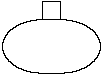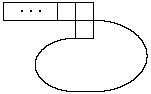Counted by b(n) Counted by c(n)

Lemma 0: For  n ≥ 2,

a(n) = a(n-1) + b(n) + c(n).                                 (1.0)
Proof: If we add a square to the right end of the top row of a horizontally convex (n-1)-omino, we obtain a horizontally convex n-omino in which the top row has at least two squares and the rightmost square in the top row is not above the leftmost square in the second row; such n-ominoes are counted by  a(n) - b(n) - c(n). Conversely, each such n-omino is obtained from a unique horizontally convex (n-1)-omino by this process. Hence  a(n) - b(n) - c(n) = a(n-1).  QED

Lemma 1: For  n ≥ 3,

c(n) = c(n-1) + a(n-2).                                      (1.1)
Proof: Let P be a polyomino counted by c(n). If P has at least three squares in the top row, we can delete the leftmost square to obtain a polyomino counted by c(n-1). If P has exactly two squares in the top row, we can delete both of them to obtain a horizontally convex (n-2)-omino. These processes are reversible, so we obtain (1.1). QED

To obtain an equation for b(n), we introduce two more functions:

Definition: If  n ≥ 1,  then:

d(n) is the number of horizontally convex n-ominoes in which the top row has exactly one square and this square is not above the rightmost square in the second row;

e(n) is the number of horizontally convex n-ominoes in which the top row has exactly one square, this square is not above the rightmost square in the second row, and the rightmost square in the second row is above the leftmost square in the third row.

The figures below suggest the approximate shapes of polyominoes counted by d(n) and e(n).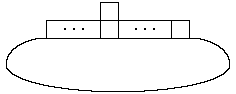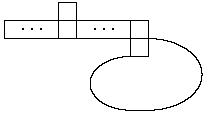Counted by d(n) Counted by e(n)

Lemma 2: For  n ≥ 2,

b(n) = a(n-1) + d(n).                                        (1.2)
Proof:  b(n) - d(n)  is the number of horizontally convex n-ominoes in which the top row has exactly one square, which is above the rightmost square in the second row. Deleting the top square from such a polyomino gives a horizontally convex (n-1)-omino. Conversely, adding a square above the rightmost square in the top row of a horizontally convex (n-1)-omino gives a polyomino counted by  b(n) - d(n).  QED

Lemma 3: For  n ≥ 3,

d(n) = b(n-1) + e(n).                                        (1.3)
Proof:  d(n) - e(n)  is the number of horizontally convex n-ominoes in which the top row has exactly one square, this square is not above the rightmost square in the second row, and the rightmost square in the second row is not above the leftmost square in the third row. (Note that this includes some n-ominoes in which there is no third row.) If we delete the rightmost square in the second row of such a polyomino, we obtain a polyomino counted by b(n-1). Conversely, adding a square to the right end of the second row of a polyomino counted by b(n-1) gives one counted by  d(n) - e(n).  (The condition n ≥ 3 is needed to ensure that a polyomino counted by b(n-1) has at least two rows.) QED

Combining Lemmas 2 and 3 gives

b(n) = b(n-1) + a(n-1) + e(n)                                (1.4)
for  n ≥ 3.

Lemma 4: For  n ≥ 2,

e(n) = e(n-1) + c(n-1).                                      (1.5)
Proof: Let P be a polyomino counted by e(n). If the top square of P is directly above the leftmost square in the second row of P, then deleting the top square gives a polyomino counted by c(n-1). Otherwise, deleting the leftmost square in the second row gives a polyomino counted by e(n-1). These operations are reversible, so (1.5) follows. QED

It is now a straightforward matter to combine equations (1.0), (1.1), (1.4), and (1.5) to obtain the Theorem: By (1.0),

a(n) - a(n-1) = b(n) + c(n)    for n ≥ 2.
Hence, for  n ≥ 3,

a(n) - 2 a(n-1) + a(n-2) = (b(n) + c(n)) - (b(n-1) + c(n-1))

= (b(n) - b(n-1)) + (c(n) - c(n-1))

= a(n-1) + e(n) + a(n-2),
by (1.1) and (1.4). Thus

a(n) - 3 a(n-1) = e(n)    for  n ≥ 3.
So, for  n ≥ 4,

a(n) - 4 a(n-1) + 3 a(n-2) = e(n) - e(n-1)

= c(n-1),
by (1.5). Finally, for  n ≥ 5,

a(n) - 5 a(n-1) + 7 a(n-2) - 3 a(n-3) = c(n-1) - c(n-2)

= a(n-3),
by (1.1). This implies the Theorem.

2. Concluding remarks

The functions b(n), c(n), d(n), and e(n) all satisfy the same recurrence as does a(n) (for sufficiently large  n),  since each can be expressed as a linear combination of a(n), a(n-1), a(n-2): In fact it is easy to show that

b(n) = 3 a(n-1) - 4 a(n-2)    for  n ≥ 4;                     (2.0)

c(n) = a(n) - 4 a(n-1) + 4 a(n-2)    for  n ≥ 4;              (2.1)

d(n) = 2 a(n-1) - 4 a(n-2)    for  n ≥ 4;                     (2.2)

e(n) = a(n) - 3 a(n-1)    for  n ≥ 3.                         (2.3)
Consequently, each satisfies an asymptotic formula like (0.1), with the same value of  v  but different values of  u.

A short table of these functions is given below. More values are given in [EIS]; see A001169 for a(n), A049219 for b(n), A049220 for c(n), A049221 for d(n), and A049222 for e(n).

n      a(n)    b(n)    c(n)    d(n)    e(n)
--      ----    ----    ----    ----    ----
1         1       1       0       1       0
2         2       1       0       0       0
3         6       3       1       1       0
4        19      10       3       4       1
5        61      33       9      14       4
6       196     107      28      46      13
7       629     344      89     148      41
8      2017    1103     285     474     130
9      6466    3535     914    1518     415
10     20727   11330    2931    4864    1329

References

[EIS] The On-Line Encyclopedia of Integer Sequences, by Neil Sloane, http://oeis.org

[K0] David A. Klarner, Some results concerning polyominoes, Fibonacci Quarterly, 3 (1965) 9-20.

[K1] David A. Klarner, Cell growth problems, Canad. J. Math., 19 (1967) 851-863.

[P] G. Pólya, On the number of certain lattice polygons, J. Comb. Theory, 6 (1969) 102-105.

[S0] Richard P. Stanley, Generating functions, in Studies in Combinatorics, edited by Gian-Carlo Rota, MAA Studies in Mathematics, vol. 17 (1978), pp. 100-141.

[S1] Richard P. Stanley, Enumerative Combinatorics, vol. 1, Cambridge Studies in Advanced Mathematics #49, Cambridge University Press, 1997.

[T] H. N. V. Temperley, Combinatorial problems suggested by the statistical mechanics of domains and of rubber-like molecules, Physical Review, ser. 2, 103 (1956) 1-16.

Received August 18, 1999. Published in Journal of Integer Sequences September 8, 1999.